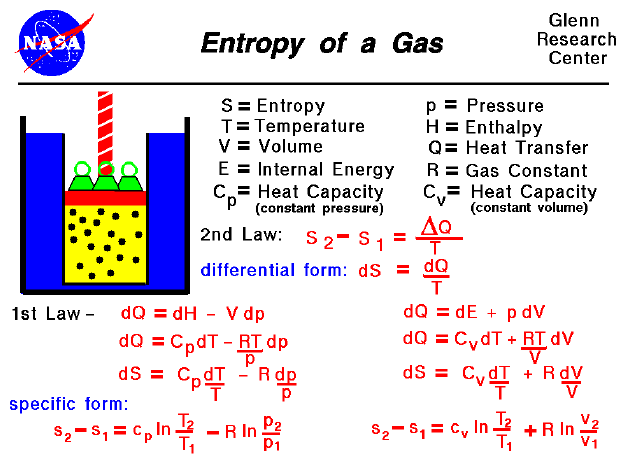A text only version of this slide is available which gives all of the flow equations.

Thermodynamics is a branch of physics that deals with the energy and work of a system. As aerodynamicists, we are most interested in thermodynamics in the study of propulsion systems and understanding high speed flows. The First Law of Thermodynamics indicates that the total energy of a system is conserved. This includes the potential and kinetic energy, the work done by the system, and the transfer of heat through the system. The Second Law of Thermodynamics indicates that, while many physical processes that satisfy the first law are possible, the only processes that occur in nature are those for which the entropy of the system either remains constant or increases. Entropy, like temperature and pressure, can be explained on both a macro scale and a micro scale. Since thermodynamics deals only with the macro scale, entropy is defined here to be the heat transfer into the system divided by the temperature.

During a thermodynamic process, the temperature of an object changes as heat is applied or extracted. A more correct definition of the entropy is the differential form that accounts for this variation.

dS = dQ / T

The change in entropy is then the inverse of the temperature integrated over the change in heat transfer. For gases, there are two possible ways to evaluate the change in entropy. The equations can be formulated in terms of the internal energy and the definition of work for a gas.

dQ = de + p dV

Using the equation of state, we can modify the second term of this equations in terms of the gas constant, the pressure, temperature, and volume.

dQ = de + R * T dV / V

The heat transfer of a gas is equal to the specific heat constant times the change in temperature; in differential form:

dQ = constant * dT

If we have a constant volume process, the formulation of the first law gives:

de = dQ = C (constant volume) * dT

Combining with the first law equation:

dQ = C (constant volume) * dT + R * T dV / V

Then the change in entropy is given by:

dS = C (constant volume) * dT / T + R * dV / V

The equations can also be formulated in terms of the enthalpy of the gas with the result:

dS = C (constant pressure) * dT / T - R * dp / p

These two relations are differential equations which we can easily integrate.

s2 - s1 = C (constant volume) * ln ( T2 / T1) + R * ln ( v2 / v1)

s2 - s1 = C (constant pressure) * ln ( T2 / T1) - R * ln ( p2 / p1)Guided Tours